Next: About this document ... Up: lab_template Previous: lab_template

Subsections

# MA 1024: Surfaces

## Purpose

The purpose of this lab is to introduce you to some of the Maple commands that can be used to plot surfaces in three dimensions.

## Getting Started

To assist you, there is a worksheet associated with this lab that contains examples and even solutions to some of the exercises. You can copy that worksheet to your home directory by going to your computer's Start menu and choose run. In the run field type:

\\filer\calclab


when you hit enter, you can then choose MA1024 and then choose the worksheet

Surf_start_C14.mw


Remember to immediately save it in your own home directory. Once you've copied and saved the worksheet, read through the background on the internet and the background of the worksheet before starting the exercises.

## Background

For functions of two variables in Cartesian coordinates, the graph is a set of pointsin three-dimensional space. For this reason, visualizing functions of two variables is usually more difficult.

One of the most valuable services provided by computer software such as Maple is that it allows us to produce intricate graphs with a minimum of effort on our part. This becomes especially apparent when it comes to functions of two variables, because there are many more computations required to produce one graph, yet Maple performs all these computations with only a little guidance from the user.

The simplest way of describing a surface in Cartesian coordinates is as the graph of a function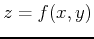over a domain, e.g. a set of points in the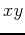plane. The domain can have any shape, but a rectangular one is the easiest to deal with. Another common, but more difficult way of describing a surface is as the graph of an equation, where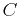is a constant. In this case, we say the surface is defined implicitly. A third way of representing a surfaceis through the use of level curves. The idea is that a plane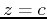intersects the surface in a curve. The projection of this curve on theplane is called a level curve. A collection of such curves for different values of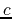is a representation of the surface called a contour plot. Similar to the idea of level curves is to look at cross sections of the surface to see what two-dimensional shape is traced, not only in theplane by letting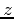be constant, but also in the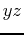plane by holding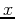constant and the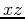plane by holding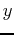constant.

## Defining Functions of Two Variables

Defining functions of two variables in Maple is very similar to the way it is done for functions of one variable - just remember the parentheses.

>f:=(x,y)->x^2+y^2;

Evaluating the function at a specific (x,y) value is easy:
>f(3,1);


## Plotting Three-dimensional Surfaces

The plot3d command is similar to the plot command except the domain has both x and y values.However, there is a lot more you can do with plot3d. First try moving the plot by clicking and dragging. Also, a menu of options will appear if you right click on the graph.

>plot3d(f(x,y),x=-2..2,y=-2..2);

Instead of using the right-click menu you can put the options into the plot command.
plot3d(f(x,y),x=-2..2,y=-2..2,scaling=constrained,axes=boxed,color=magenta);


## Cross Sections

The easiest way to get cross sections (parallel to the x-y plane) is to use the Maple command contourplot which is included in the package plots. The following command will show 15 cross sections using z-values that the computer will choose.

>with(plots):
>contourplot(f(x,y),x=-2..2,y=-2..2,contours=15);

Thefollowing command will show 4 cross sections using z-values that you choose.
>contourplot(f(x,y),x=-2..2,y=-2..2,contours=[-1,0,1,2]);

In the above commands the z-value was held constant thus giving a two-dimensional plot. You can hold x or y constant to get a cross section perpendicular to the y-z or x-z plane. To get these cross sections use the plot command.
>plot(f(5,y),y=-2..2,labels=[y,z]);

Note how easy it is to hold the x (or y) constant when you have entered a function, f(5,y). Also note that the axes were labeled to emphasize what the two remaining variables are. Next is an example of four contours parallel to the x-z plane.
>plot({f(x,-1),f(x,0),f(x,1),f(x,2)},x=-2..2,labels=[x,z]);


## Exercises

1. Generate a surface plot with boxed axes and contour plot with 22 contours for the following function on the given domain: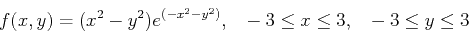A
Describe the difference in proximity between the contour lines in the regions where the surface plot has a steep incline compared to where the surface plot is almost flat?
B
What does the contour plot look like in regions where the surface plot has local extrema?
C
Estimate the local maximum and minimum by rotating the 3-dimensional plot and state your estimations in text.
D
Evaluate the funtion at all four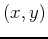coordinate pairs for approximately where the contourplot indicates a local extrema to see if your estimates were close.

2. Create a contour plot for the functionfor thevalues -2, -1, -1/2, 1/2, 1, 2 twice; using Maple's contourplot command and using the implicitplot command. Use the domain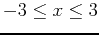,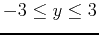and use the option ,numpoints=3000 in both commands.

3. For the surface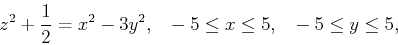Plot 2 cross section curves in each coordinate plane (use the option ,scaling=constrained and ,numpoints=3000 in the-plane) and state what shapes are graphed. Identify the type or three dimensional shape of the quadric surface, ie. a sphere, cylinder, cone, elliptic cone, paraboloid, elliptic parabaloid, ellipsoid, hyperboloid of one sheet, hyperboloid of two sheets, elliptic hyperboloid of one or two sheets, or a hyperbolic parabaloid (saddle). Once you have determined the shape of the surface, supply a three dimensional plot to support your conclusion.Next: About this document ... Up: lab_template Previous: lab_template
Dina J. Solitro-Rassias
2014-01-05